### Home > CALC > Chapter 5 > Lesson 5.1.4 > Problem5-44

5-44.
1. A "CHAIN" OF FUNCTIONS
Remember that composite functions are made by "chaining" simpler functions together. For example, f(x) = 2x2 + 3 is made by chaining the functions h(x) = 2x2 and g(x) = x + 3.

2. It can be useful to show this idea with an arrow diagram:

3.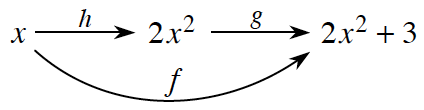4. Above is an arrow diagram for h followed by g, which would be written f(x) = g(h(x)). (Notice that the "inner" function is applied first.)

5. Let h(x) = tan x + 5 and g(x) = (x + 3)2. Draw an arrow diagram and determine the composite function for each f(x) below. Simplifying is not required. Homework Help ✎

1. f(x) = g(h(x))

2. f(x) = h(g(x))

3. f(x) = g(g(h(x)))

4. f(x) = h(g(g(x)))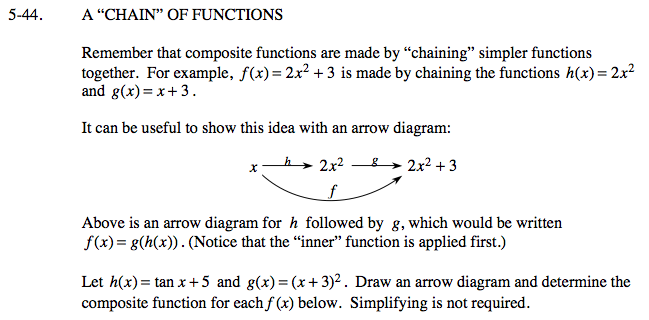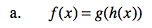$x\overset{h}{\rightarrow}\text{tan } x+5\overset{g}{\rightarrow}((\text{tan }x+5)+3)^{2}=(\text{tan }x+8)^{2}$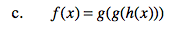Refer to example above and hint in part (a).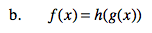Refer to example above and hint in part (a).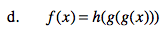Refer to example above and hint in part (a).